# Physics

?

## Static Electricity

an insulator can be charged by friction through the transfer of electrons, the material gaining the electrons becomes negatively charged whilst the material losing electrons is left with an equal positive charge.

like charges repel and opposite charges attract

if a balloon has a negative charge and a wall has no charge, the balloon will repel the electrons in the wall, leaving a postive charged wall. which it will then be attracted to. this is called induction and the charge is an induced charge. the same happens with a charged comb picking up paper.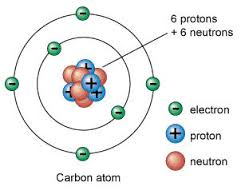1 of 27

## Dangers and Uses of Static Electricity

charge (negative) builds up as shoes walk across carpet= negatively charged person. if you touch a metal door electrons flow from you to the object. causing an electric shock. meaning you are disharged.

static can also build up in clouds causing a huge spark between the clouds and the ground. the lightning we see is a stream of charged particles in the atmosphere.

static electricity is only dangerous when it is likely to cause a spark. if a conducting path can be provided then there is no danger of a spark.

when refuelling aircraft, a bonding line (metal wire) is used to connect the aircraft to Earth before it is refuelled = discharged

it can also be useful:

object is given a negative charge, paint is given a positive charge, paint particles spread out giving a more even coat and is also attracted to the negative surface so none is wasted.

2 of 27

## Electric Currents

an electric current is the rate of flow of charge

a current in a metal is the flow of electrons.

charge(coulomb) = current (ampere) x time (second)

Q=Ixt

cells and batteries supply a d.c current (movement of charge in one direction only)

3 of 27

## Current and Voltage

an ammeter is placed in series with a component to measure the current in Amps

the current is always conserved at a junction (equal split)

the current of a circuit depends on the potential difference (voltage) of the source

a voltmeter is placed in parallel with a component to measure the voltage in Volts

the p.d is the energy transferred per unit charge passed and therefore the volt is a joule per coulomb.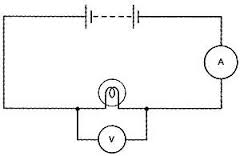4 of 27

## Changing Resistances

changing the resistance in a circuit changes the current of the circuit and this can be done using a variable resistor.

potential difference(volt) = current (ampere) x resistance (ohm)

V = I x R

filament lamps:

• as they get hotter, resistance increases
• a higher p.d makes them hotter

diodes:

• conduct electricity in one direction only
• resistance of a LDR decreases as it gets lighter

thermistors:

• resistance gets higher as it gets colder
5 of 27

## Transferring Energy

when there is an electric current in a resistor there is an energy transfer which heats the resistor

the energy transfer is the result of collisions between electrons and ions in the lattice

an advantage would be the heating affect as it can be used in electrical fires and kettles.

a similar thing happens with all components. in the case of a motor, wasted energy would be the heat produced . some electrical appliances could produce too much waste energy and become too hot to touch or create a fire (eg. a plug), although most plugs are fitted with fuses which will melt and break the circuit if too high.

electrical power(Watt) = Current(ampere) x Potential Difference(volt)
P = I x V

energy transferred(Joule) = current(ampere) x p.d(volt) x time(second)
E = I x V x t

6 of 27

## Vectors and Velocity

vector quantities:

• displacement
• velocity- speed in a stated direction
• momentum
• acceleration
• force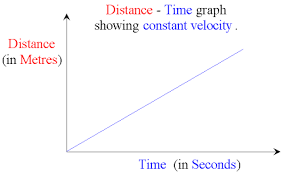speed(m/s) = distance(m) / time(s)

7 of 27

## Acceleration

most moving things do not stay at a constant velocity. they speed up or slow down all the time, this change in velocity is called acceleration - it has magnitude and direction.

if a moving object changes it's velocity or direction, it is accelerating.

acceleration(m/s)2 = change in velocity(m/s) / time taken
a = (v-u)
t

8 of 27

## Velocity-time Graphs

you can use velocity-time graphs to:

• compare accelerations from gradients qualitatively
• calculate the acceleration from the gradientyou can use them to calculate the distance travelled by using the area between the graph line and the time axis.

9 of 27

## Forces

free-body diagram: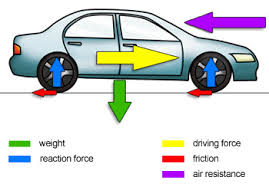when two bodies interact, the forces they exert on eachother are equal in size and opposite in direction.

they are called action and reaction forces.

10 of 27

## Resultant Forces

if the resultant force acting on a body is zero, it will remain at rest or continue to move at a constant velocity.

if it is not zero, then it will accelerate in the direction of the resultant force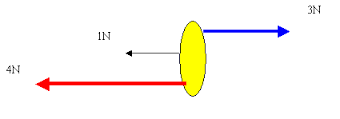11 of 27

## Forces and Acceleration

the resultant force acting on an object produces an acceleration but depends on:

• size of the resultant force
• mass of the object

force(Newton) = mass(kg) x acceleration(m/s2)

F = m x a

12 of 27

## Terminal Velocity

weight(Newton) = mass(kg) x gfs(N/kg)
W = m x g

in a vacuum, all falling bodies accelerate at the same rate.

• when an object falls through an atmosphere, air resistance increases with increasing speed
• air resistance increases until it is equal in size to the falling weight
• when the two forces are balanced, the acceleration is zero and terminal velocity is reached13 of 27

## Stopping Distances

the stopping distance of a vehicle is the sum of the thinking distance and the braking distance

factors that can affect stopping distance are:

• mass of the vehicle
• speed of the vehicle
• drivers reaction time
• state of the vehicles brakes
• friction between tyre and road surface

factors that can affect thinking distance are:

• drivers concentration
• inhibitions lowered by alcohol ect.
14 of 27

## Momentum

momentum(kg m/s) = mass(kg) x velocity (m/s)

momentum is a vector quantitty which means that direction matters

the conservation of linear momentum:

when a moving object collides with another object, the total momentum of the objects before and after the collision stays the same

15 of 27

## Momentum and Safety

the faster the momentum of an object changes, the greater the force on the object. safety measures involve reducing the rate of change of momentum in order to reduce the forces e.g.

• bubble wrap
• seat belt
• crumple zone
• air bag

force(Newton) = change in momentum(kg m/s)
time(s)

16 of 27

## Work and Power

work done(J) = force(N) x distance moved in direction of force(m)
E = F x d

energy transferred(Joule) = work done (Joule)

power is the rate of doing work and is measured in Watts and one watt is equal to one joule per second (J/s)

Power(W) = work done(J)
time taken(s)

when work done stopping a vehicle is the braking force multiplied by the braking distance, when stopped, all the vehicle's kinetic energy has been removed. meaning the work done is equal to the initial kinetic energy

17 of 27

## Potential and Kinetic Energy

GPE(J) =mass(kg) x GFS(N/kg) x vertical height(m)
GPE = m x g x h

KE(J) = mass(kg) x velocity(m/s)2
2

when energy is transferred, the total amount always stays the same. when an object with GPE falls down, the amount of KE it has at the instant of hitting the ground is equal to it's initial GPE.

18 of 27

atoms may lose or gain electrons in which case it will gain a charge and become an ion.

ionising radiation has enough energy to cause atoms to lose electrons.

a radioactive substance has an unstable nucleus, which decays to become more stable by losing losing energy when certain types of ionising radiation are emmited from the nucleus (alpha, beta and gamma). the nuclei decay randomly as it is a random process.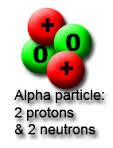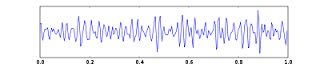alpha-                       beta-                                                gamma-
bad penetration       moderate penetraton                       good penetration
very ionising            moderate ionisation                          low ionisation

19 of 27

## Nuclear Reactions

Nuclear reactions can be a source of energy: fission, fusion and radioactive decaythe fission of U-235 also releases energy

an uncontrolled nuclear reaction as shown above has nothing to absorb the two extra neutrons and therefore is absorbed by other nuclei, making them split up ect. eg. an atomic bomb
a controlled reaction is when another material absorbs the extra neutrons.

fission involves splitting atoms and fusion involves joining atoms

20 of 27

## Nuclear Power

a chain reaction is controlled in a nuclear reactor as moderators slow down neutrons and control rods absorb neutrons.

the thermal/ heat energy from the chain reaction is converted into electrical energy in a nuclear power station:

the thermal energy is transferred into a coolant(usually water at high pressure), which is pumped through a reactor, the super-heated water is pumped to a 'heat exchanger' where it is used to produce steam, the steam turns a turbine, which turns a generator which transfers kinetic energy to electrical energy.

however the products of nuclear fission are radioactive and the radioactive waste builds up in the reactor core.

21 of 27

## Fusion

nuclear fusion is the creation of larger nuclei from smaller nuclei, accompanied by a release of energy. it is the energy source for stars.

nuclear fission has to happen at high temperatures and pressures due to electrostatic repulsion of protons, they have to be moving very quickly and be very close together.

meaning that the temperature inside a fusion reactor must be very high. this high temperature and pressure is very hard to sustain.

theories such as cold fusion are not accepted until they have been validated by the scientific community.

22 of 27

## Changing Ideas

ionising radiation can be dangerous as it can cause tissue damage and possible mutations and precautions need to be taken.

scientists have changed their ideas of radioactivity over time including the awareness of hazards associated with radioactive sources23 of 27

## Nuclear Waste

high leve waste (HLW)- transported in thick concrete/steel containers to absorb radiation(50years)
intermediate level waste (ILW)- stored in concrete/steel containers(none disposed yet)
low level waste (LLW)- compacted and buried in special landfill sites

• doesn't emit carbon dioxide
• energy used to make fuel rods requires emmiting carbon dioxide
• has to be stored for tens of thousands of years
• risk of leaking into the environment
• risk of accidents like Chernobyl24 of 27

## Half-life

the activity of radioactive sources decreases over time, the unit used to measure the activity of a radioactive isotope is the becquerel ( Bq ).

the half-life of a radioactive isotope is the time it takes for half the undecayed nuclei to decay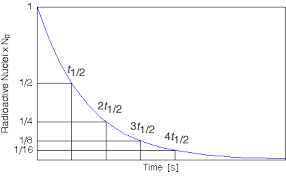25 of 27

background radiation is ionising radiation at a low level, from space and naturally radioactive substances in the environment.

regional variations in the UK are caused in particular by radon gas

high-energy charged particles stream out of the Sun and other stars ect. they are called cosmic rays and are a form of ionising radiation, many are stopped by the upper atmosphere but some still reach the Earth's surface.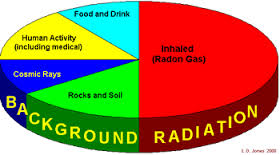26 of 27

• sterilisation of equipment - gamma
• diagnosis and treatment of cancer (radiotherapy) - gamma
• household smoke alarmssmoke particles absorb alpha particles and the current decreases• tracing/gauging thickness (beta)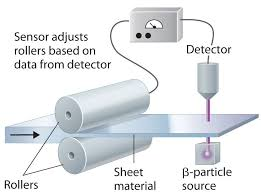27 of 27